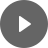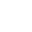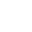11.2 Loans - StudyNinja
Select Page

HSC Standard Maths Resources

Browse: 1. Home  »  11. Depreciation & Loans »  11.2 Loans

11.2 Loans

In this section, we will talk about reducing-balance loan. Unlike in ‘Investment’ section where simple interest is applied on the loan and the interest payments don’t change over the life of the loan, reducing-balance loan applies interest on the balance of the loan outstanding at the start of each repayment period.Each of these will be looked at in turn below through examples.

Calculating amount owing on a loan

At a basic level, you will be given loan’s principal, interest rate and repayment information and would be required to work out loan balance at a given period.

Following formulas would be helpful for this:

Balance @ t = (Balance @ t – 1) + (Interest @ t) – Repayment for period

Interest @ t = (Balance @ t – 1) × interest rate for period

Example 1

Harry borrowed \$28,000 to buy a car with interest of 3.99% p.a. and monthly repayments of \$515. What is the amount owing after 3 months?

Looking up loan repayments from table

This should generally be straight-forward question where a table will be given, and values need to be looked up to do some loan related calculations.

Example 2

Following table shows monthly repayment schedule for \$1 borrowed on a loan. What is monthly repayment for a 5-year loan of \$15,000 with interest rate of 3.5%p.a.?Graph of loan

Example 3

The following chart shows loan balance over months. Answer the following questions:

1. How much loan is taken?
2. How long the loan is borrowed for?
3. Between which months half of the loan is paid off?Get access to 20 Mock Exams with over 700 exam-style questions for HSC Standard Maths.

You might find the following videos helpful related to this section:3F Reducing Balance Loans (1 of 4)

by Mr Bodgers (click to view channel)

Explains how a reducing balance loan works.3F Reducing Balance Loans (2 of 4)

by Mr Bodgers (click to view channel)

Solves a reducing balance loan question using a table that can be used to calculate the monthly repayments.3F Reducing Balance Loans (3 of 4)

by Mr Bodgers (click to view channel)

Solves a reducing balance loan question using a table that can be used to calculate the monthly repayments.3F Reducing Balance Loans (4 of 4)

by Mr Bodgers (click to view channel)

Solves a reducing balance loan question using using a graph that shows two different repayment optionsThe following is the type of questions you can expect in exam:

Study notes of this section and other resources can be accessed here:

Browse: 1. Home  »  11. Depreciation & Loans »  11.2 Loans